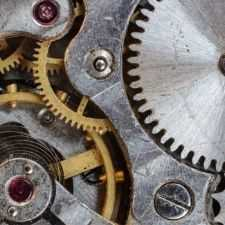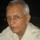Mechanical
Community of Mechanical Engineers: Get help about everything related to mechanical engineering from fellow mechanical engineers.
832 Members
Join this group to post and comment.robo_warrior • Jun 18, 2012

# Quantum mechanical problem

Quantum mechanics thought to be the toughest subject in universe but i have thought of a paradox in its very core and want to know what do you think about it :
The Heisenberg uncertainty principle states that one cannot predict both the position and velocity of an electron in an atom because :
*if you want to detect an electron you need to have a particle smaller than electron itself.
*and the only particle known smaller than e- is photon.
*but photons of wavelength of dimension of e- are highly energetic.
*if one tries to detect position of electron , photon striking the electron changes its original configuration or state(knocks the e- out of atom).
*what i have thought is if we accelerate the atom to 99.99% of the speed of light then electron's mass will become super huge and then if we use the photon of that dimension it won't affect the electron configuration much as the change in momentum will be less
And change in position and velocity will also be lessIt is not that simple.
We cannot use terms of classical mechanics to explain quantum mechanics.
What is implied is that if the position of an electron is fixed in an atom then there are are an infinite number of momentum values valid for that location. Contrarily, if you fix a momentum value thentherte are a number of locations of the electron that will fit that value.
Speeding the whole atom to near speed of light requires a frame of reference with which that speed is increased. If the measurement has to be made from that (relatively) stationary frame of reference How is the measurement to be made? In any case if the whole atom is moving the electron itself will still be behaving as if the atom is stationary.
I know that this is confusing. The problem is the statistical nature of quantum mechanics.
https://www.aip.org/history/heisenberg/p08b.htm